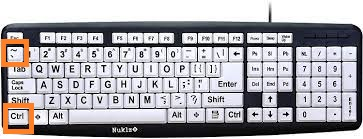Google Spreadsheet software is a great tool where we can create magic with our datasets. We use various formulas and functions to structure, organize, and perform mathematical operations in Google Sheets. But, when we are using functions or formulas in Google Sheets, we just the result not the formula or function used. However, the spreadsheet also allows us to see what formula or function is used in a particular cell with the help of its in-built feature.

Let’s consider the following dataset. Here I have various formulas in each cell of the spreadsheet.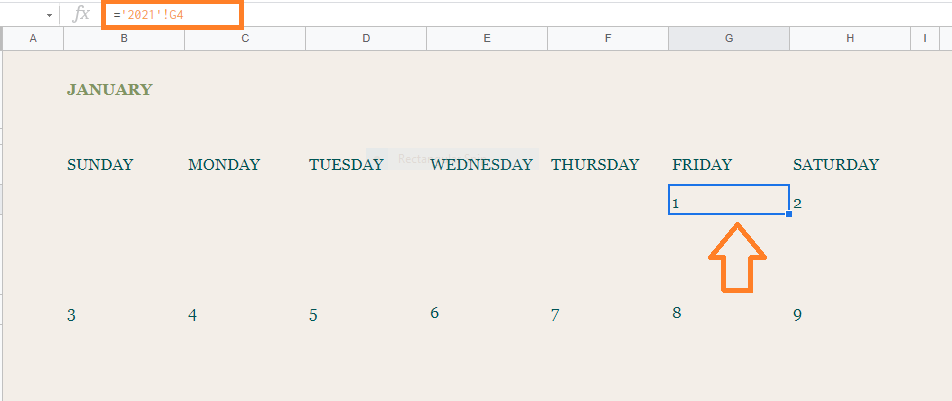Now follow the steps as listed below to show formulas instead of values:

• Step 1: Open the Google Spreadsheet where you would like to show formulas instead of values.
• Step 2: Click on the “View” tab in the menubar.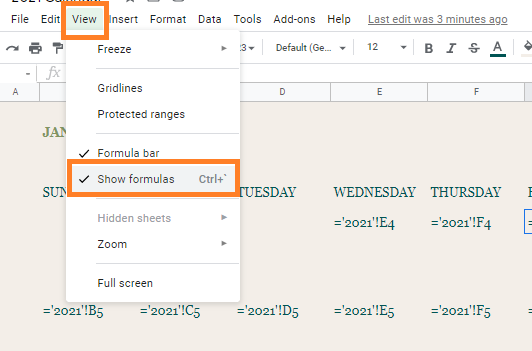• Step 3: Now select “Show Formulas” from the drop-down menu.
• Step 4: This will automatically show formulas instead of values in Google Sheets.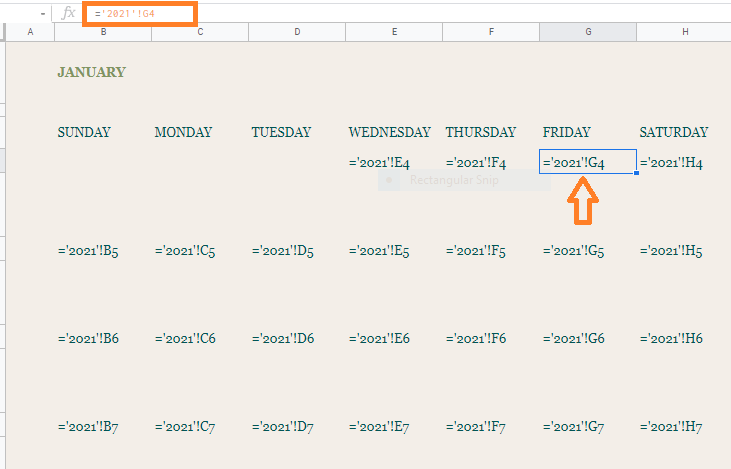This strategy can help you grasp what’s going on in a spreadsheet that you are unfamiliar with. Looking at someone else’s work is also a great approach to learn how to use formulas and analysis tools.

This view is very useful when analyzing a workbook that appears to be returning incorrect values. This allows you to see all of the formulas at once rather than having to click on each cell to figure out what’s going on.

In order to convert back formulas to values follow the steps as listed below:

• Step 2: Now uncheck “Show Formulas” from the drop-down menu.

This will show values now instead of formulas.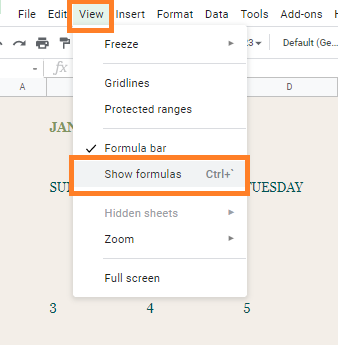How to Show both Formula and Values at the Same Time?

In Google Sheets, we can see both formulas and values at the same time. To know how to do this, follow the steps as listed below:

• Step 1: Select the cell where you would like to view the formula.
• Step 2: Double click the cell to see the detailed functions of the cell.
• Step 3: Click on the “?” icon next to the cell to bring up the pop-up value preview. Alternatively, you can also “F9” key to enable the pop-up value preview.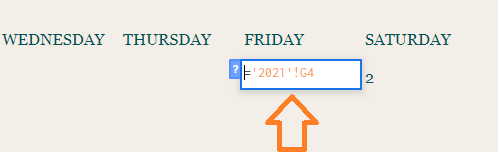These tricks come in handy when trying to understand what’s going on with a particular formula in the cell. So you can fix formulas and see all the information at once.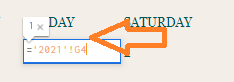How to Show Formula by Converting it to Text?

This method can be adopted if you want the values to display as formulas permanently.

In order to compel Sheets to show instead than process a formula, you only need to place the formula in apostrophe before it.

Google Sheets doesn’t include a right-click menu or other formatting choices to control cell formatting, unlike other cell formatting approaches. Changing the formula in the cell is the only option.

Now follow the steps listed below to show formulas by converting them to text.

• Step 1: Select the cell where you would like to show formulas instead of values.
• Step 2: Now simply add an apostrophe before the formula.
• Step 3: Press the “Enter” button. The apostrophe will be hidden in Google Sheets and the only formula will be displayed as shown below.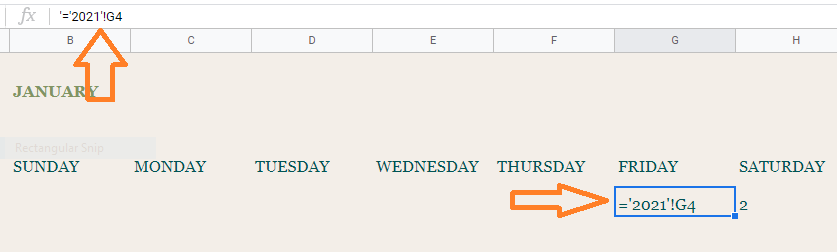Your formula will be displayed instead of the value when the apostrophe symbol is used. Apostrophes in one cell will replace all cell contents with that cell’s value if you try to move the value across a range. When you add an apostrophe to a calculation, Sheets will not automatically modify the formulas.

How to Show formulas instead of values in Google Sheets using Keyboard Shortcut?

This is one of the easiest methods where you don’t have to execute multiple steps. The steps to achieve “shows formulas instead of values” are given below: